NEET  >  31 Years NEET Previous Year Questions: Current Electricity - 3

# 31 Years NEET Previous Year Questions: Current Electricity - 3

Test Description

## 30 Questions MCQ Test Physics Class 12 | 31 Years NEET Previous Year Questions: Current Electricity - 3

31 Years NEET Previous Year Questions: Current Electricity - 3 for NEET 2023 is part of Physics Class 12 preparation. The 31 Years NEET Previous Year Questions: Current Electricity - 3 questions and answers have been prepared according to the NEET exam syllabus.The 31 Years NEET Previous Year Questions: Current Electricity - 3 MCQs are made for NEET 2023 Exam. Find important definitions, questions, notes, meanings, examples, exercises, MCQs and online tests for 31 Years NEET Previous Year Questions: Current Electricity - 3 below.
Solutions of 31 Years NEET Previous Year Questions: Current Electricity - 3 questions in English are available as part of our Physics Class 12 for NEET & 31 Years NEET Previous Year Questions: Current Electricity - 3 solutions in Hindi for Physics Class 12 course. Download more important topics, notes, lectures and mock test series for NEET Exam by signing up for free. Attempt 31 Years NEET Previous Year Questions: Current Electricity - 3 | 30 questions in 60 minutes | Mock test for NEET preparation | Free important questions MCQ to study Physics Class 12 for NEET Exam | Download free PDF with solutions
 1 Crore+ students have signed up on EduRev. Have you?
31 Years NEET Previous Year Questions: Current Electricity - 3 - Question 1

### The masses of the three wires of copper are inthe ratio of 1 : 3 : 5 and their lengths are in theratio of 5 : 3 : 1. The ratio of their electricalresistance is 

Detailed Solution for 31 Years NEET Previous Year Questions: Current Electricity - 3 - Question 1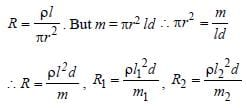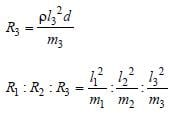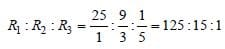31 Years NEET Previous Year Questions: Current Electricity - 3 - Question 2

### Two batteries of emf 4 V and 8V with internal resistance 1 Ω and 2 Ω are connected in a circuit with a resistance of 9 Ω as shown in figure. The current and potential difference between the points P and Q are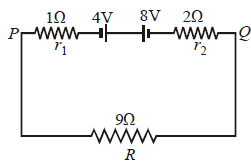Detailed Solution for 31 Years NEET Previous Year Questions: Current Electricity - 3 - Question 2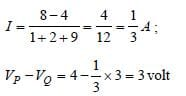31 Years NEET Previous Year Questions: Current Electricity - 3 - Question 3

### n equal resistors are first connected in seriesand then connected in parallel. What is the ratioof the maximum to the minimum resistance ?

Detailed Solution for 31 Years NEET Previous Year Questions: Current Electricity - 3 - Question 3

In series, Rs= nR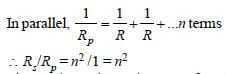31 Years NEET Previous Year Questions: Current Electricity - 3 - Question 4

Forty electric bulbs are connected in series across a 220 V supply. After one bulb is fused the remaining 39 are connected again in series across the same supply. The illumination will be     

Detailed Solution for 31 Years NEET Previous Year Questions: Current Electricity - 3 - Question 4

Since, the voltage is same for the two
combinations, therefore

H ∝ 1/R.

Hence, the
combination of 39 bulbs will glow more.

31 Years NEET Previous Year Questions: Current Electricity - 3 - Question 5

A current of 2 A, passing through a conductorproduces 80 J of heat in 10 seconds. Theresistance of the conductor in ohm is 

Detailed Solution for 31 Years NEET Previous Year Questions: Current Electricity - 3 - Question 5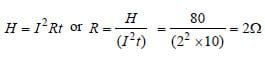31 Years NEET Previous Year Questions: Current Electricity - 3 - Question 6

Two identical batteries each of e.m.f 2V andinternal resistance 1Ω are available to produceheat in an external resistance by passing a currentthrough it. The maximum power that can bedeveloped across R using these batteries is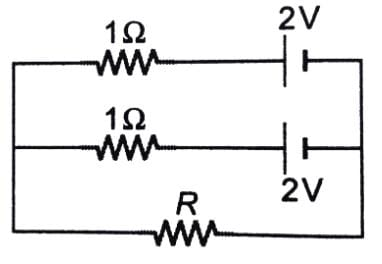Detailed Solution for 31 Years NEET Previous Year Questions: Current Electricity - 3 - Question 6

For maximum current, the two batteries
should be connected in series. The current
will be maximum when external resistance is
equal to the total internal resistance of cells
i.e. 2Ω. Hence power devloped across the
resistacne R will be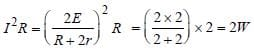31 Years NEET Previous Year Questions: Current Electricity - 3 - Question 7

You are given several identical resistances eachof value R = 10Ω and each capable of carrying amaximum current of one ampere. It is required tomake a suitable combination of these resistancesof 5Ω which can carry a current of 4 ampere. Theminimum number of resistances of the type Rthat will be required for this job is 

Detailed Solution for 31 Years NEET Previous Year Questions: Current Electricity - 3 - Question 7

To carry a current of 4 ampere, we need four
paths, each carrying a current of one ampere.

Let r be the resistance of each path. These
are connected in parallel. Hence, their
equivalent resistance will be r/4. According
to the given problem r/4 = 5 or r = 20Ω
For this propose two resistances should be
connected. There are four such
combinations. Hence, the total number of
resistance = 4 × 2 = 8.

31 Years NEET Previous Year Questions: Current Electricity - 3 - Question 8

In the network shown in the Fig, each resistance is 1Ω. The effective resistance between A and B  is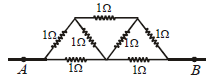Detailed Solution for 31 Years NEET Previous Year Questions: Current Electricity - 3 - Question 8

At A current is distributed and at B currents
are collected. Between A and B, the
distribution is symmetrical. It has been
shown in the figure. It appears that current
in AO and OB remains same. At O, current i4
returns back without any change. If we
detach O from AB there will not be any
change in distribution.
Now, CO & OD will be in series hence its
total resistance = 2Ω
It is in parallel with CD, so, equivalent
resistance =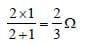This equivalent resistance is in series with
AC & DB, so, total resistance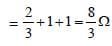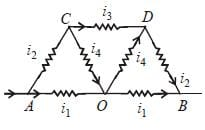Now 8/3 Ω is parallel to AB, that is, 2Ω so
total resistance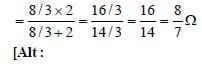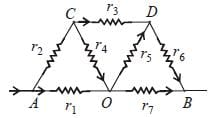Between C & D, the equivalent resistance is
given by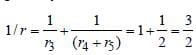Equivalent resistance along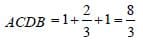∴ Effective resistance between A and B is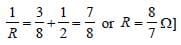31 Years NEET Previous Year Questions: Current Electricity - 3 - Question 9

Faraday’s laws are consequence of conservationof 

Detailed Solution for 31 Years NEET Previous Year Questions: Current Electricity - 3 - Question 9

Faraday’s laws are based on the conversion
of electrical energy into mechanical energy;
which is in accordance with the law of
conservation of energy

31 Years NEET Previous Year Questions: Current Electricity - 3 - Question 10

The velocity of charge carriers of current (about1 amp) in a metal under normal conditions is ofthe order of 

Detailed Solution for 31 Years NEET Previous Year Questions: Current Electricity - 3 - Question 10 The velocity of charge carriers of current 1 ampere in ametal under normal conditions is of the order of a fraction ofmm/sec
31 Years NEET Previous Year Questions: Current Electricity - 3 - Question 11

Direct current is passed through a coppersulphate solution using platinum electrodes. Theelements liberated at the electrodes are 

Detailed Solution for 31 Years NEET Previous Year Questions: Current Electricity - 3 - Question 11

In the electrolysis of CuSO4, oxygen is
liberated at anode and copper is deposited
at cathode.

31 Years NEET Previous Year Questions: Current Electricity - 3 - Question 12

Three resistances each of 4 Ω are connected toform a triangle. The resistance between any twoterminals is 

Detailed Solution for 31 Years NEET Previous Year Questions: Current Electricity - 3 - Question 12

The two resistances are connected in series
and the resultant is connected in parallel with
the third resistance.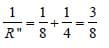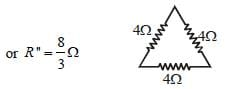31 Years NEET Previous Year Questions: Current Electricity - 3 - Question 13

Current through 3 Ω resistor is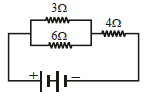0.8 amp., then potential drop through 4Ω resistor is           

Detailed Solution for 31 Years NEET Previous Year Questions: Current Electricity - 3 - Question 13

Voltage across 3 Ω resistance = 3 × 0.8 = 2.4V
This voltage is the same across 6 Ω
resistance. Hence current through this
resistance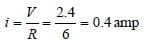Total current in the circuit
= 0.8 + 0.4 = 1.2 amp
Voltage across 4 Ω resistance
= 4 × 1.2 = 4.8 volts

31 Years NEET Previous Year Questions: Current Electricity - 3 - Question 14

A 4 μF capacitor is charged to 400 volts andthen its plates are joined through a resistance of1k Ω. The heat produced in the resistance is           

Detailed Solution for 31 Years NEET Previous Year Questions: Current Electricity - 3 - Question 14

The energy stored in the capacitor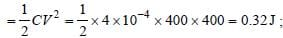This energy will be converted into heat in
the resistor.

31 Years NEET Previous Year Questions: Current Electricity - 3 - Question 15

In the circuit shown in Fig, the current in 4 Ω resistance is 1.2 A. What is the potential difference between B and C.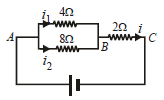Detailed Solution for 31 Years NEET Previous Year Questions: Current Electricity - 3 - Question 15

The potential difference across 4Ω resistance
is given by

V = 4 × i1 = 4 × 1.2 = 4.8 volt

So, the potential across 8Ω resistance is also
4.8 volt.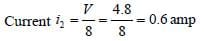Current in 2Ω resistance i = i1 + i2
∴ i = 1.2 + 0.6 = 1.8 amp
Potential difference across 2Ω resistance
VBC = 1.8 × 2 = 3.6volts

31 Years NEET Previous Year Questions: Current Electricity - 3 - Question 16

A heating coil is labelled 100 W, 220 V. The coil iscut in half and the two pieces are joined in parallelto the same source. The energy now liberatedper second is 

Detailed Solution for 31 Years NEET Previous Year Questions: Current Electricity - 3 - Question 16

Power of heating coil = 100 W and voltage
(V) = 220 volts. When the heating coil is cut
into two equal parts and these parts are
joined in parallel, then the resistance of the
coil is reduced to one-fourth of the previous
value. Therefore energy liberated per second
becomes 4 times i.e., 4 × 100 = 400 J.

31 Years NEET Previous Year Questions: Current Electricity - 3 - Question 17

If a wire of resistance R is melted and recasted tohalf of its length, then the new resistance of thewire will be 

Detailed Solution for 31 Years NEET Previous Year Questions: Current Electricity - 3 - Question 17

Initial resistance (R1) = R; Initial length is
1and final length ( 2) = 0.5 . Volume of a
wire = . A. Since the volume of the wire
remains the same after recasting, therefore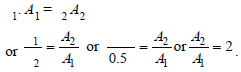We also know that resistance of a wire (R)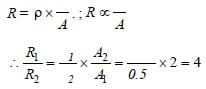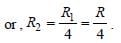[Alt : When wires are drawn from same
volume but with different area of
cross-section, then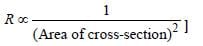31 Years NEET Previous Year Questions: Current Electricity - 3 - Question 18

Two wires of the same metal have same length,but their cross-sections are in the ratio 3:1. Theyare joined in series. The resistance of thickerwire is 10Ω. The total resistance of thecombination will be 

Detailed Solution for 31 Years NEET Previous Year Questions: Current Electricity - 3 - Question 18

Length of each wire = ; Area of thick wire
(A1) = 3A; Area of thin wire (A2) = A and resistance of thick wire (R1) = 10 Ω

Resistance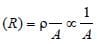(if is constant)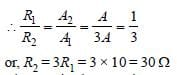The equivalent resistance of these two
resistors in series
= R1 + R, = 30 + 10 = 40Ω.

31 Years NEET Previous Year Questions: Current Electricity - 3 - Question 19

If the resistance of a conductor is 5 Ω at 50ºCand 7Ω at 100ºC, then the mean temperature coefficient of resistance (of the material) is

Detailed Solution for 31 Years NEET Previous Year Questions: Current Electricity - 3 - Question 19

Explanation : We know that RT = R0(1+αT)  where RT = resistance at temp T, R0 = resistance at temp T = 0oC and α= temperature coefficient of resistance.

Here, 5 = R0(1+α.50)...(1)

and 7 = R0(1+α.100)...(2)

(2)/(1)

⇒ 7/5 = (1+100α)/(1+50α)

7+350α = 5+500α

150α=2

⇒α=2/150

=0.013/oC

31 Years NEET Previous Year Questions: Current Electricity - 3 - Question 20

What will be the equivalent resistance of circuitshown in figure between two points A and D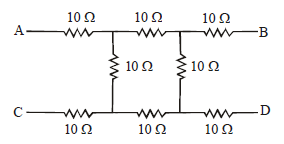Detailed Solution for 31 Years NEET Previous Year Questions: Current Electricity - 3 - Question 20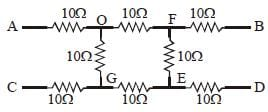Equivalent Circuit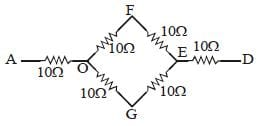Equivalent Resistance of circuit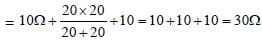31 Years NEET Previous Year Questions: Current Electricity - 3 - Question 21

If a negligibly small current is passed through awire of length 15 m and of resistance 5Ω havinguniform cross-section of 6 × 10–7 m2 , thencoefficient of resistivity of material, is 

Detailed Solution for 31 Years NEET Previous Year Questions: Current Electricity - 3 - Question 21

Given : Length of wire (l) = 15m
Area (A) = 6 × 10–7 m2
Resistance (R) = 5Ω.
We know that resistance of the wire material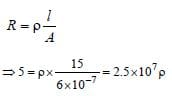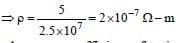31 Years NEET Previous Year Questions: Current Electricity - 3 - Question 22

Kirchoff’s first law, i.e. ∑i = 0 at a junction, dealswith the conservation of [1992, 1997]

Detailed Solution for 31 Years NEET Previous Year Questions: Current Electricity - 3 - Question 22

We know from the Kirchhoff 's first law that
the algebraic sum of the current meeting at
any junction in the circuit is zero (i.e. ∑i = 0)
or the total charge remains constant.
Therefore, Kirchhoff's first law at a junction
deals with the conservation of charge

31 Years NEET Previous Year Questions: Current Electricity - 3 - Question 23

A (100 W, 200 V) bulb is connected to a 160Vpower supply. The power consumption would be    

Detailed Solution for 31 Years NEET Previous Year Questions: Current Electricity - 3 - Question 23

Power = 100 W, Voltage = 200 V

Resistance of bulb =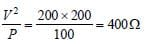When bulb is applied across 160 V,
Current in bulb = 160/400 A

Power consumption =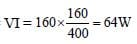31 Years NEET Previous Year Questions: Current Electricity - 3 - Question 24

There are three copper wires of length and cross sectional area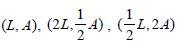.   Inwhich case is the resistance minimum?   

Detailed Solution for 31 Years NEET Previous Year Questions: Current Electricity - 3 - Question 24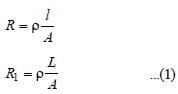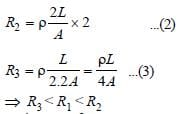31 Years NEET Previous Year Questions: Current Electricity - 3 - Question 25

The current in the following circuit is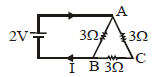Detailed Solution for 31 Years NEET Previous Year Questions: Current Electricity - 3 - Question 25

Resistance of ACB, R' = 3Ω + 3Ω = 6Ω.
For net resistance between A and B; R' = 6Ω
and 3Ω are in parallel.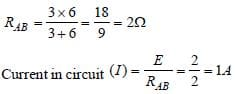31 Years NEET Previous Year Questions: Current Electricity - 3 - Question 26

Three equal resistors connected across a sourceof e.m.f. together dissipate 10 watt of power.What will be the power dissipated in watts if thesame resistors are connected in parallel acrossthe same source of e.m.f.?      

Detailed Solution for 31 Years NEET Previous Year Questions: Current Electricity - 3 - Question 26

In series, Equivalent resistance = 3R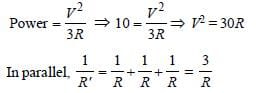∴ Equivalent resistance R' = R/3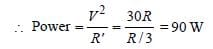31 Years NEET Previous Year Questions: Current Electricity - 3 - Question 27

If nearly 105 coulombs liberate 1 gm-equivalentof aluminium, then the amount of aluminium(equivalent weight 9), deposited throughelectrolysis in 20 minutes by a current of 50 amp.will be 

Detailed Solution for 31 Years NEET Previous Year Questions: Current Electricity - 3 - Question 27

m = Zit, 9 = Z × 105, Z= 9 × 10–5
Again,
m = Zit = 9 × 10–5 × 50 × 20 × 60 = 5.4 gm

31 Years NEET Previous Year Questions: Current Electricity - 3 - Question 28

A 5ºC rise in temperature is observed in aconductor by passing a current. When thecurrent is doubled the rise in temperature will beapproximately 

Detailed Solution for 31 Years NEET Previous Year Questions: Current Electricity - 3 - Question 28

Since H ∝ I2 , doubling the current will
produce 4 times heat. Hence, the rise in
temperature will also be 4 times i.e., rise in
temperature = 4 × 5 = 20ºC.

31 Years NEET Previous Year Questions: Current Electricity - 3 - Question 29

The resistance of a discharge tube is 

Detailed Solution for 31 Years NEET Previous Year Questions: Current Electricity - 3 - Question 29

In discharge tube the current is due to flow
of positive ions and electrons. Moreover,
secondary emission of electrons is also
possible. So V-I curve is non-linear; hence
resistance is non-ohmic.

31 Years NEET Previous Year Questions: Current Electricity - 3 - Question 30

The current (I) in the given circuit is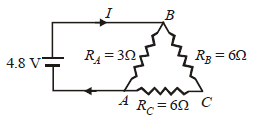Detailed Solution for 31 Years NEET Previous Year Questions: Current Electricity - 3 - Question 30

In circuit, RB and RC are in series, so, Rs = 6 +
6 = 12Ω. This 12 Ω resistance is in parallel
with RA = 3 Ω,
So, equivalent resistance of circuit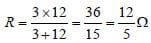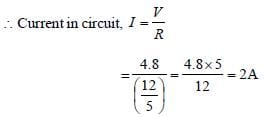## Physics Class 12

157 videos|452 docs|213 tests
 Use Code STAYHOME200 and get INR 200 additional OFF Use Coupon Code
Information about 31 Years NEET Previous Year Questions: Current Electricity - 3 Page
In this test you can find the Exam questions for 31 Years NEET Previous Year Questions: Current Electricity - 3 solved & explained in the simplest way possible. Besides giving Questions and answers for 31 Years NEET Previous Year Questions: Current Electricity - 3, EduRev gives you an ample number of Online tests for practice

## Physics Class 12

157 videos|452 docs|213 tests

### How to Prepare for NEET

Read our guide to prepare for NEET which is created by Toppers & the best Teachers